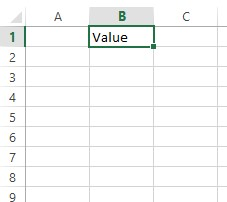# Activate Worksheet in Excel in VBA

This article helps us understand how to activate Worksheet in Excel in VBA with the help of some examples.

## Activate Worksheet in Excel in VBA

When working in VBA with Excel files, there are multiple sheets. If we want to run the same process or function on all the sheets in single or multiple Excel files, we need to make another sheet active before running any function.

It takes time to write code for each sheet separately again and again.

For this purpose, VBA provides a method, `Activate`, that can be used to make any sheet active while working on Excel.

Let’s go through an example in which we will use VBA `activate` worksheet method to make the sheet an active sheet. This method is widely used when we write VBA macros.

We can use either the name or the number of the Worksheet. The best way is to use the sheet name.

``````Sub activateNewSheet()
Worksheets("Sheet2").Activate
'Worksheets(2).Activate
End Sub
``````

This will make the `Sheet2` or the second sheet in the Excel file an active worksheet.

### `ActiveSheet` Object in VBA

Imagine if our current sheet is active or if we have activated another sheet in our code with the help of the `Activate` method. We want to mention the currently active sheet to perform some tasks.

We can easily mention it with the help of an object that is specifically made for the active sheet as `ActiveSheet`.

If we are working on graphs or charts and have forgotten which sheet is active right now, we can use the following code to directly mention the currently active sheet in VBA. We can use two options to perform the function on the active sheet, as shown below.

``````Sub addValueActiveSheet()
ActiveSheet.Range("B1")="Value"
Range("B1")="Value"
End Sub
``````

Output:As you can see from the above code, we have used two methods, and both gave the same result because it doesn’t matter whether we mention the active sheet.

Once we set any sheet as an active sheet, the next code will automatically be implemented on the same sheet.

### Set `ActiveSheet` in VBA

Now, let’s discuss setting the `ActiveSheet` in VBA. It is helpful to mention the Active Sheet in Excel VBA by setting it into a variable.

We can set and assign an active sheet to an object and use that object to mention the active sheet where ever we want. We can mention it at any place during the procedure.

The below code will print some value at range `B1` of the Active Sheet.

``````Sub setActivSheet()

Dim active As Worksheet
Set active = ActiveSheet

active.Range("B1") = "Value"
End Sub
``````

Output:Write for us
DelftStack articles are written by software geeks like you. If you also would like to contribute to DelftStack by writing paid articles, you can check the write for us page.

## Related Article - VBA Worksheet

• Set Worksheets in VBA